# Transfer function

for a linear stationary control system (an automatic control system)

The Laplace transform of the response to a unit pulse function (delta-function)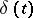with zero conditions at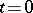(this response is called the weighting function, the pulse transfer function or the pulse characteristic). An equivalent definition is that the transfer function is the ratio of the Laplace transforms (see Operational calculus) for the output and input signals with zero initial data. The transfer function is a rational-fractional function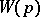of the complex variable; it is the coefficient in the linear relation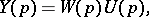(1)

relating the Laplace transform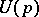of the input of the system (the action, the control)and the Laplace transform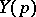of the output (response, reaction)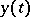with zero initial conditions. In control theory, relation (1) is represented graphically as: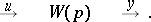For example, let the control system be described by a linear ordinary differential equation with constant coefficients: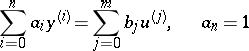(2)

(in an actual system,usually). Then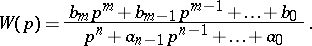(3)

The same expression can be obtained if one uses the operator form of (2) and the differentiation operator,to define the transfer function as the ratio of the input operator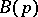to the eigenoperator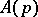; the transfer function (3) of (2) has the following interpretation: If one selects the control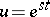, whereis a complex number such that, then the linear inhomogeneous equation (2) has the particular solution.

The transfer function should not be confused with the step response, which is the response of the system to the unit step function: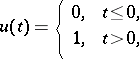with zero initial conditions.

The transfer function is one of the basic concepts in the theory of linear stationary control systems. It is independent of the control actions imposed on the system and is governed only by the parameters of the system itself, thus providing a dynamical characteristic itself. The function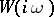of a pure-imaginary argument plays a particular part in control theory; it is called the amplitude-phase, or frequency, characteristic. The concept of a transfer function has been extended also to linear control systems of other types (matrix, non-stationary, discrete, with distributed parameters, etc.).